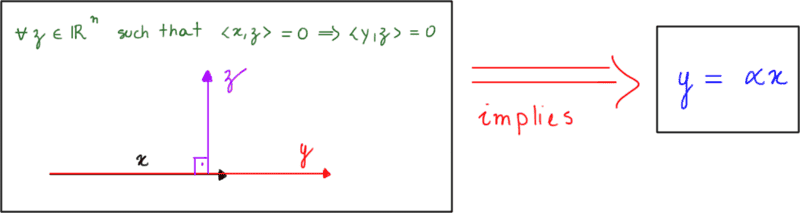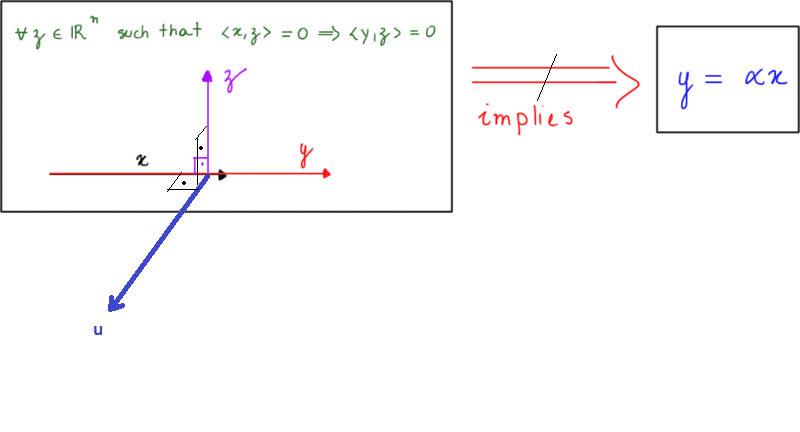# Inner product - Analysis in Rn problem

• Onezimo Cardoso
In summary: We can see this in the following way:Given a vector ##x_1## we can write it as the sum of two vectors, one being the projection of ##x_1## onto the vector ##x## and the other being the projection of the same vector ##x_1## onto a vector orthogonal to ##x##. In other words we can write ##x_1 = c_1x + c_2(x_1 - c_1x)##.

## Homework Statement

Let ##x,y \in \mathbb{R^n}## not null vectors. If for all ##z \in \mathbb{R^n}## that is orthogonal to ##x## we have that ##z## is also orthogonal to ##y##, prove that ##x## and ##y## are multiple of each other.

## Homework Equations

We can use that fact that ##<x , y-\frac{<x,y>}{|x|^2}x> = 0##

## The Attempt at a Solution

If ##x=y## the result follow directly. So let us suppose that ##x\neq y##.
Using the fact that ##<x , y-\frac{<x,y>}{|x|^2}x> = 0## and the hypothesis we can affirm that ##<y , y-\frac{<x,y>}{|x|^2}x> = 0##.
Further, by the fact that ##<x , y-\frac{<x,y>}{|x|^2}x> = 0## and ##<y , y-\frac{<x,y>}{|x|^2}x> = 0## we have:
$$<x , y-\frac{<x,y>}{|x|^2}x> = <y , y-\frac{<x,y>}{|x|^2}x>$$
$$\Rightarrow <x , y-\frac{<x,y>}{|x|^2}x> - <y , y-\frac{<x,y>}{|x|^2}x> = 0$$
$$\Rightarrow <x - y , y-\frac{<x,y>}{|x|^2}x> =0 \quad (*)$$

Until here everything seems great. But now...

Once ##x\neq y## , second the source I got this solution, by ##(*)## we can affirm that:
$$y-\frac{<x,y>}{|x|^2}x = 0$$
$$\Rightarrow y = \alpha x$$
Where ##\alpha = \frac{<x,y>}{|x|^2}x ##

But the fact is if we have ##<x,y>=0## not necessarily we must have ##x=0## or ##y=0##.
Can anyone see if I could not see a relevant fact in the argument or someone have another look for this problem?

Last edited:
Onezimo Cardoso said:

## Homework Statement

Let ##x,y \in \mathbb{R^n}## not null vectors. If for all ##z \in \mathbb{R^n}## that is orthogonal to ##x## we have that ##z## is also orthogonal to ##y##, prove that ##x## and ##y## are multiple of each other.

## Homework Equations

We can use that fact that ##<x , y-\frac{<x,y>}{|x|^2}x> = 0##

## The Attempt at a Solution

If ##x=y## the result follow directly. So let us suppose that ##x\neq y##.
Using the fact that ##<x , y-\frac{<x,y>}{|x|^2}x> = 0## and the hypothesis we can affirm that ##<y , y-\frac{<x,y>}{|x|^2}x> = 0##.
Further, by the fact that ##<x , y-\frac{<x,y>}{|x|^2}x> = 0## and ##<y , y-\frac{<x,y>}{|x|^2}x> = 0## we have:
$$<x , y-\frac{<x,y>}{|x|^2}x> = <y , y-\frac{<x,y>}{|x|^2}x>$$
$$\Rightarrow <x , y-\frac{<x,y>}{|x|^2}x> - <y , y-\frac{<x,y>}{|x|^2}x> = 0$$
$$\Rightarrow <x - y , y-\frac{<x,y>}{|x|^2}x> =0 \quad (*)$$

Until here everything seems great. But now...

Once ##x\neq y## , second the source I got this solution, by ##(*)## we can affirm that:
$$y-\frac{<x,y>}{|x|^2}x = 0$$
$$\Rightarrow y = \alpha x$$
Where ##\alpha = \frac{<x,y>}{|x|^2}x ##

But the fact is if we have ##<x,y>=0## not necessarily we must have ##x=0## or ##y=0##.
Can anyone see if I could not see a relevant fact in the argument or someone have another look for this problem?

Why can you assume
Using the fact that ##<x , y-\frac{<x,y>}{|x|^2}x> = 0##
##<x , y-\frac{<x,y>}{|x|^2}x>= <x,y>-<x,\alpha x>=<x,y>=0\,,## so you start with orthogonal vectors and end up with orthogonal vectors. Nothing happened in between.

Which results about basis and / or orthogonal basis do you know? E.g. you could write ##\mathbb{R}^n=\mathbb{R}x\oplus \mathbb{R}^{n-1}=\mathbb{R}y\oplus \mathbb{R}^{n-1}## with orthogonal subspaces. Your condition is:
$$\forall \, z\in \mathbb{R}^n\, \text{ with } \,\langle x , z\rangle = 0 \, : \, (\,\langle y,z \rangle = 0 \Longrightarrow x \sim y\,)$$
The fact, that it holds for all such ##z## has somehow to be used. It cannot work with a single one.

fresh_42 said:
Why can you assume
##<x , y-\frac{<x,y>}{|x|^2}x>= <x,y>-<x,\alpha x>=<x,y>=0\,,## so you start with orthogonal vectors and end up with orthogonal vectors. Nothing happened in between.

Which results about basis and / or orthogonal basis do you know? E.g. you could write ##\mathbb{R}^n=\mathbb{R}x\oplus \mathbb{R}^{n-1}=\mathbb{R}y\oplus \mathbb{R}^{n-1}## with orthogonal subspaces. Your condition is:
$$\forall \, z\in \mathbb{R}^n\, \text{ with } \,\langle x , z\rangle = 0 \, : \, (\,\langle y,z \rangle = 0 \Longrightarrow x \sim y\,)$$
The fact, that it holds for all such ##z## has somehow to be used. It cannot work with a single one.

But let me repharse the proof above and try to express myself better in order to show that the only problem with the proof above (at least for me) is the fact that ##(*)## and ##x-y \neq 0## implies that ##y-\frac{<x,y>}{|x|^2}x = 0##.

Let's begin:

Let us suppose that $$\forall z \in \mathbb{R}^n ; <x,z>=0 \Rightarrow <y,z>=0$$
Particularly, if we consider ##z_0=y-\frac{<x,y>}{|x|^2}x## , we have direclty that ##<x,z_0> = 0##. So by the hypothesis written just above we must have that ##<y,z_0>=0##, i.e. ##<y,y-\frac{<x,y>}{|x|^2}x>=0##... (And this proof goes on until stuck in ##(*)##)...

...
Notice that geometrically this really makes sense:#### Attachments

Last edited:
Onezimo Cardoso said:
But let me repharse the proof above and try to express myself better in order to show that the only problem with the proof above (at least for me) is the fact that ##(*)## and ##x-y \neq 0## implies that ##y-\frac{<x,y>}{|x|^2}x = 0##.

Let's begin:

Let us suppose that $$\forall z \in \mathbb{R}^n ; <x,z>=0 \Rightarrow <y,z>=0$$
This is not what you have. From ##\forall z \in \mathbb{R}^n ; <x,z>=0## it follows immediately that ##x=0## because the inner product is not degenerated and thus ##\langle x,z \rangle = \langle 0,z\rangle = 0## is trivial and always true. And because it is a tautology, it will not help you.
Particularly, if we consider ##z_0=y-\frac{<x,y>}{|x|^2}x## , we have direclty that ##<x,z_0> = 0##. So by the hypothesis written just above we must have that ##<y,z_0>=0##, i.e. ##<y,y-\frac{<x,y>}{|x|^2}x>=0##... (And this proof goes on until stuck in ##(*)##)...
Why can you assume ##\langle x,z_0\rangle=\langle x,y\rangle =0\,?## If you start with orthogonal ##x,y## they barely can be linear dependent.

I'm afraid you will have to use an entire basis, preferably orthogonal, but I don't know whether you can already assume it to exist. I would have placed your exercise behind the Gram-Schmidt algorithm in a book, however, I don't know your book and therefore not what you already can assume as given. That's what our section 2 of the template is meant for!

•Onezimo Cardoso#### Attachments

•Onezimo Cardoso
The condition should read: Let ##\mathfrak{x},\mathfrak{y} \in \mathbb{R}^n -\{0\}##.
\begin{align*}
&\text{IF } &\mathfrak{y} \perp U:=\{\,\mathfrak{z}\,|\,\langle \mathfrak{x},\mathfrak{z}\rangle=0\,\} = (\mathbb{R}\mathfrak{x})^\perp \cong \mathbb{R}^{n-1} \\[6pt]
&\text{THAT IS } &\langle \mathfrak{y},\mathfrak{z}\rangle =0 \,\,\forall \, \mathfrak{z}\in U \subsetneq \mathbb{R}^n \\[6pt]
&\text{THEN } & \mathfrak{y} \sim \mathfrak{x} \\[6pt]
&\text{THAT IS } & \exists \,\alpha \in \mathbb{R}-\{0\} \, : \, \mathfrak{y}=\alpha \cdot \mathfrak{x}
\end{align*}
or for short, you have to show ##(\operatorname{span}\{\,x\,\}^\perp)^\perp = \operatorname{span}\{\,x\,\}\,.##

I hope I haven't confused the letters, but it was you who started with fraktur. Anyway, what you can see is, that a single ##z## is irrelevant, and you have to use any resp. all from the subspace ##U## of all vectors perpendicular to ##x##, which is not the entire vector space, but a subspace of codimension one. This was the reason I asked for the propositions you already know. Otherwise you'll have to repeat the Gram-Schmidt procedure in a way, or use induction on ##n## which is always a good idea when dealing with finite dimensional vector spaces. But in any case, the eintire ##U## is needed and the fact that it has codimension one, for otherwise we will always find a direction which we haven't used yet, and which is perpendicular to all of them; as shown in my modification of your drawing.

•Onezimo Cardoso
I think Fresh may have been alluding to this, but I'll add that there's a very nice way to do this using Projectors, in particular a well chosen real symmetric ##\mathbf P## that obeys ##\mathbf P^2 = \mathbf P## would make this a 2-4 line exercise.

On the other hand, if OP has never heard terms like idempotent or Projector, then ignore this comment.

do you have any dimension theory? specifically do you know that the orthogonal complement of an r dimensional subspace has dimension n-r? if so then both vectors x and y belong to the same one dimensional subspace under your hypothesis. i.e. (xperp)perp = y(perp)perp is one dimensional and contains both x and y.

•Onezimo Cardoso
mathwonk said:
do you have any dimension theory? specifically do you know that the orthogonal complement of an r dimensional subspace has dimension n-r? if so then both vectors x and y belong to the same one dimensional subspace under your hypothesis. i.e. (xperp)perp = y(perp)perp is one dimensional and contains both x and y.

Great mathwonk! I'm just arranging a complete solution using your argument in order to help others that could reach this question.

Let ##x,y \in \mathbb{R}^n## not null vectors.
Let us suppose that for all ##z \in \mathbb{R}^n## which is orthogonal to ##x## it is true that ##z## is ortogonal to ##y##.
Let us considerer the orthogonal subspaces of ##x## and ##y## respectively:
$$(x^{\perp})= \{z \in \mathbb{R}^n ; <z,x>=0\}$$
$$(y^{\perp})=\{z \in \mathbb{R}^n ; <z,y>=0\}$$
From our hypothesis we have that ##(x^{\perp}) \subset (y^{\perp})##. But ##dim(x^{\perp})=dim(y^{\perp})##, then ##(x^{\perp}) = (y^{\perp})##.
Let ##S=[x,y]## be the span of ##\{ x,y \}##. Let ##(S^{\perp})## be the orthogonal complement of ##S##.
From our hypothesis we have ##(x^{\perp}) = (y^{\perp})=(S^{\perp})##.
Then ##dim((S^{\perp}))=n-1##.
But
$$dim(S)+dim((S^{\perp}))=n$$
$$\Rightarrow dim(S)=1$$
Therefore, ##x## and ##y## are linear dependent.

•Delta2